Next: Summary Up: RMS Deviation Previous: RMS Deviation

## Defining the Centre of the Volume

In the above formula it is assumed that the volume of integration is centred about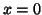. If this is not the case (when the origin of the world coordinates is not at the centre of the brain) then the matrixcan be simply modified to include a general centre.

That is, let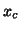be the desired centre, and define a new coordinate(33)

This new coordinate is now zero when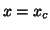, so that the above derivation is valid for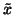. Furthermore, this translation can be expressed in matrix form (using homogeneous coordinates) as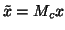where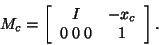Therefore,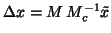, giving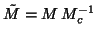. This implies that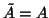and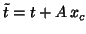so that(34)

Mark Jenkinson 2003-02-11